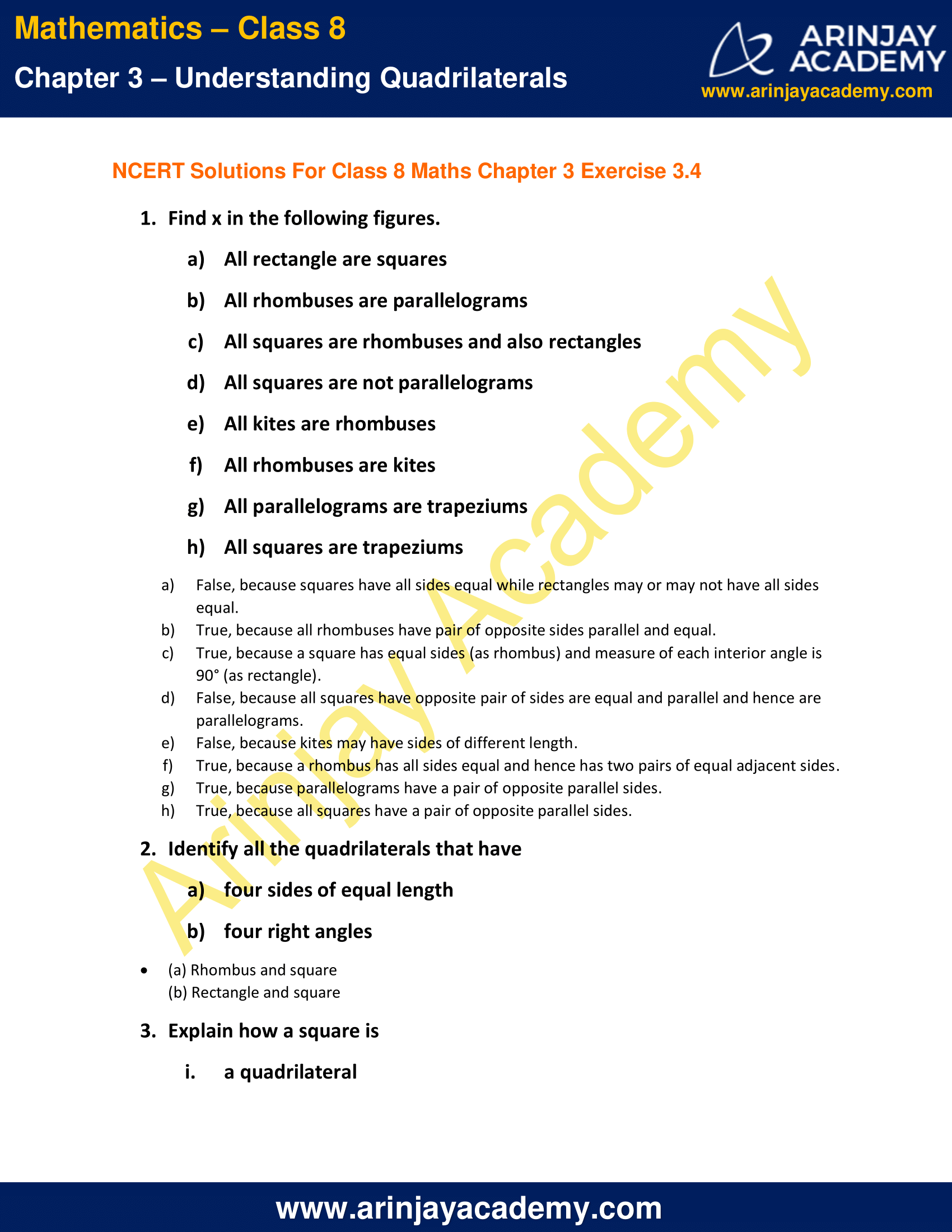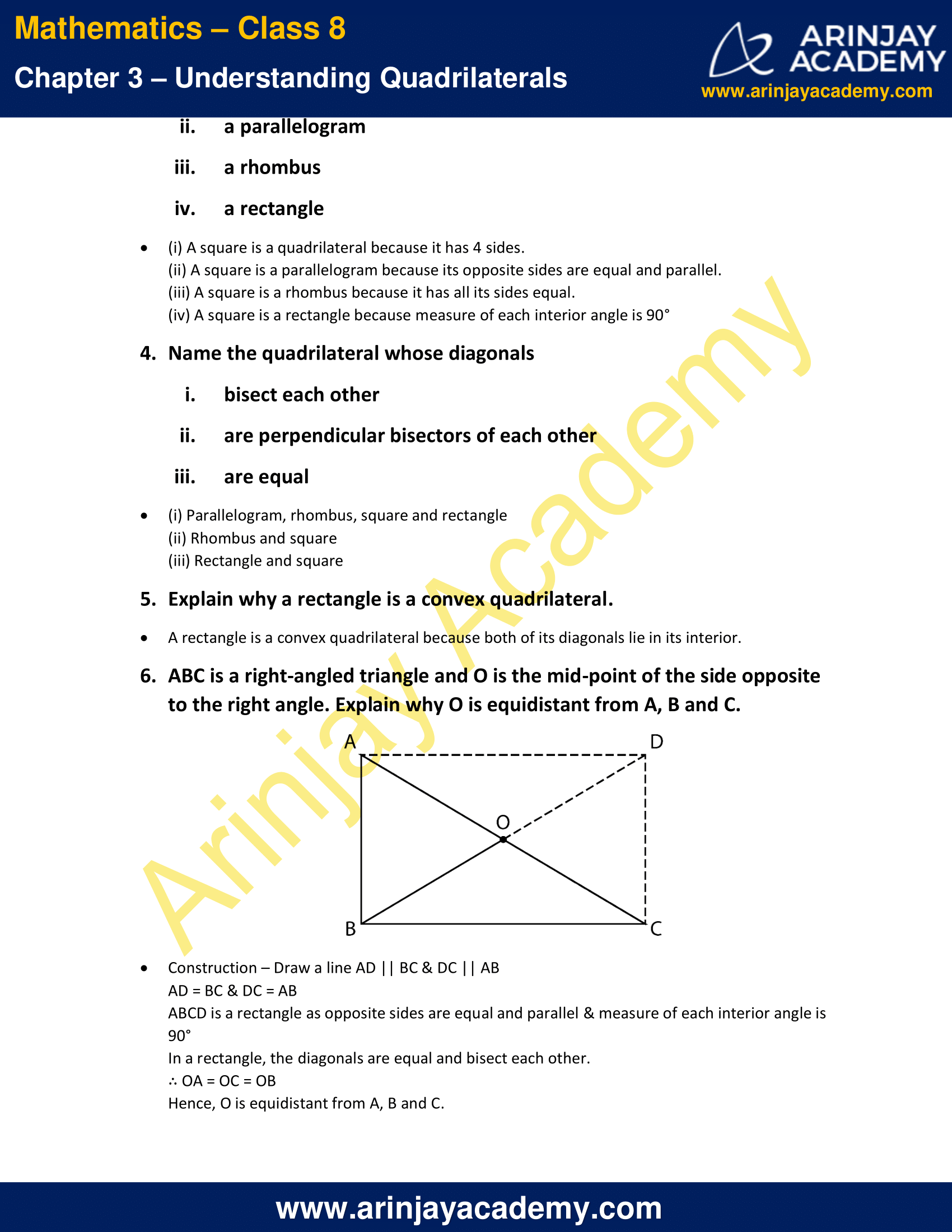# NCERT Solutions for Class 8 Maths Chapter 3 Exercise 3.4

NCERT Solutions for Class 8 Maths Chapter 3 Exercise 3.4 – Understanding Quadrilaterals, has been designed by the NCERT to test the knowledge of the student on the following topics :

Some Special Parallelograms

• Rhombus
• A rectangle
• A square1. Find x in the following figures.

a) All rectangle are squares
b) All rhombuses are parallelograms
c) All squares are rhombuses and also rectangles
d) All squares are not parallelograms
e) All kites are rhombuses
f) All rhombuses are kites
g) All parallelograms are trapeziums
h) All squares are trapeziums

Solution:

a) False, because squares have all sides equal while rectangles may or may not have all sides equal.
b) True, because all rhombuses have pair of opposite sides parallel and equal.
c) True, because a square has equal sides (as rhombus) and measure of each interior angle is 90° (as rectangle).
d) False, because all squares have opposite pair of sides are equal and parallel and hence are parallelograms.
e) False, because kites may have sides of different length.
f) True, because a rhombus has all sides equal and hence has two pairs of equal adjacent sides.
g) True, because parallelograms have a pair of opposite parallel sides.
h) True, because all squares have a pair of opposite parallel sides.

2. Identify all the quadrilaterals that have

a) four sides of equal length
b) four right angles

Solution:

(a) Rhombus and square
(b) Rectangle and square

3. Explain how a square is

ii. a parallelogram
iii. a rhombus
iv. a rectangle

Solution:

(i) A square is a quadrilateral because it has 4 sides.
(ii) A square is a parallelogram because its opposite sides are equal and parallel.
(iii) A square is a rhombus because it has all its sides equal.
(iv) A square is a rectangle because measure of each interior angle is 90°

4. Name the quadrilateral whose diagonals

i. bisect each other
ii. are perpendicular bisectors of each other
iii. are equal

Solution:

(i) Parallelogram, rhombus, square and rectangle
(ii) Rhombus and square
(iii) Rectangle and square

5. Explain why a rectangle is a convex quadrilateral.

Solution:

A rectangle is a convex quadrilateral because both of its diagonals lie in its interior.

6. ABC is a right-angled triangle and O is the mid-point of the side opposite to the right angle. Explain why O is equidistant from A, B and C.Solution:

Construction – Draw a line AD || BC & DC || AB
AD = BC & DC = AB

ABCD is a rectangle as opposite sides are equal and parallel & measure of each interior angle is 90°

In a rectangle, the diagonals are equal and bisect each other.
∴ OA = OC = OB

Hence, O is equidistant from A, B and C.

The next Exercise for NCERT Solutions for Class 8 Maths Chapter 4 Exercise 4.1 – Practical Geometry can be accessed by clicking here